Function Repository Resource:

# FunctionAudiolize

Illustrate the behavior of a real-valued function in the form of sound

Contributed by: Rauan Kaldybayev
 ResourceFunction["FunctionAudiolize"][y,{x,xmin,xmax},T] makes an "audiolization" of y as a function of x from xmin to xmax, with the resulting audio having a length of T seconds.

## Details and Options

ResourceFunction["FunctionAudiolize"] outputs a piece of audio such that the frequency at a particular moment is determined by the value of the plotted function for the corresponding value of the independent variable. The volume is constant.
ResourceFunction["FunctionAudiolize"] only accepts real-valued functions.
Frequency as a function of time, f(t), is determined by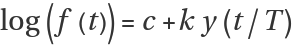, where y(x) is the plotted function and k>=0,T>0. The higher the value of y, the higher the frequency. If y is negative and has a large absolute value, the frequency is low.
ResourceFunction["FunctionAudiolize"] can be useful to mathematicians/math enthusiasts who want to enhance their intuition about a particular function by converting it to audio; this especially applies to audials, people who perceive information better through hearing.
An audiolization can often be more intuitive than a regular plot. The ability to perceive functions through sound can potentially be tremendously helpful to sight-impaired people who want to learn math, but do not have access to the traditional teaching techniques that rely heavily on visual input.
ResourceFunction["FunctionAudiolize"] supports the following options:
 "BaselineFrequency" 440 average frequency of the audiolization, in hertz "MinFrequency" 120 a lower bound below which the frequency is not allowed to fall, in hertz "MaxFrequency" 1910 an upper bound above which the frequency is not allowed to rise, in hertz "TimeStep" 0.01 time steps for discretization of the plotting domain SampleRate 8000 SampleRate of ResourceFunction["FunctionAudiolize"]; determines the quality of sound
The frequency cannot surpass "MinFrequency" and "MaxFrequency", but it is not obliged to touch both of these bounds. The frequency generally touches exactly one of those limits, either the upper or the lower one.
The mean value of log(f(t)) over the length of the audio is bound to be equal to Log["BaselineFrequency"].
ResourceFunction["FunctionAudiolize"] uses the built-in function Play to make sounds. It computes the frequency as a function of time by rescaling the plotted function, finds the sound pressure as a piecewise sine function, and uses Play.

## Examples

### Basic Examples (2)

Make a 10-second audiolization of the function f = Exp[-x/30]Cos[x] for x from 0 to 20π and compare to the regular plot. The swings in pitch reflect the oscillations of the function, and the decrease in the magnitude of these swings over time reflects the decaying exponential factor:

 In:=Out=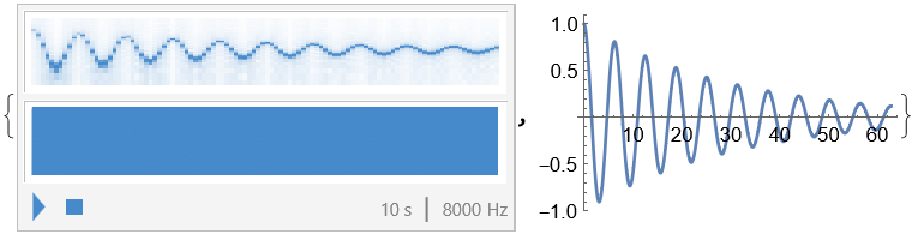Use the function 1/x, where the audiolization starts with a high note and then drops down, resembling a whining dog:

 In:=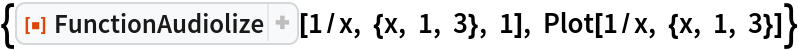Out=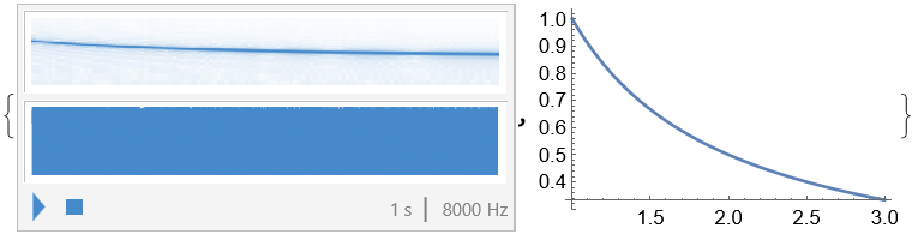### Scope (2)

While FunctionAudiolize does not directly support complex-valued functions, it is possible to plot the absolute value and phase as those are real functions:

 In:=Out=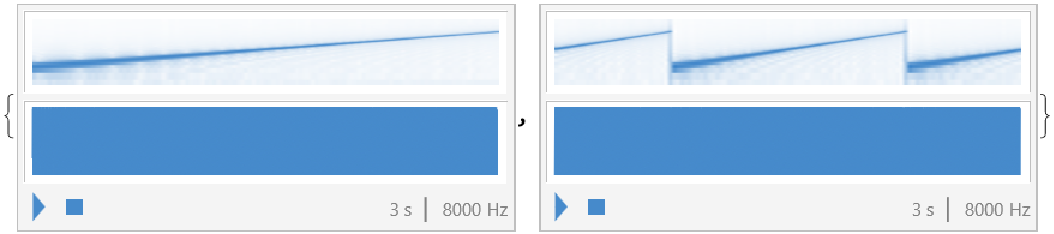One can instead work with the real and imaginary parts:

 In:=Out=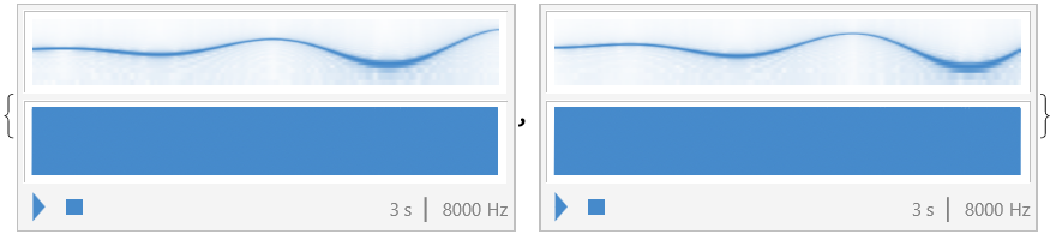### Options (5)

#### BaselineFrequency (1)

Change the baseline frequency (which is the mean frequency over the duration of the audio) from the default value of 440 Hz to 800 Hz:

 In:=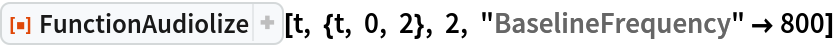Out=#### MinFrequency (1)

In addition to the requirement that the mean frequency should have a certain value, the frequency also cannot fall below or exceed the given bounds, set by default to 120 and 1910 Hz. Changing the lower limit to 360 Hz decreases the frequency range:

 In:=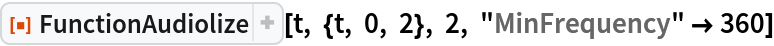Out=#### SampleRate (1)

Decrease the sample rate of FunctionAudiolize to decrease the computation time:

 In:=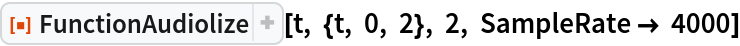Out=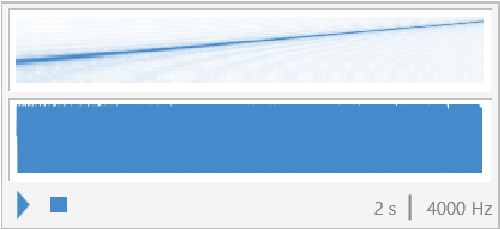#### TimeStep (2)

Increasing the time steps means that the domain will be split into fewer regions, which reduces the computation time at the cost of resolution:

 In:=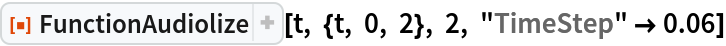Out=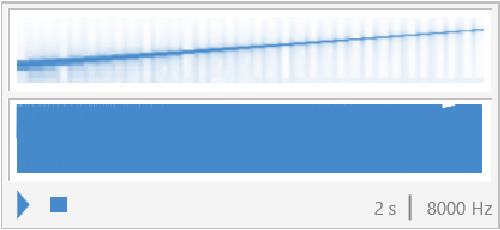For faster-changing functions, this effect can become significant:

 In:=Out=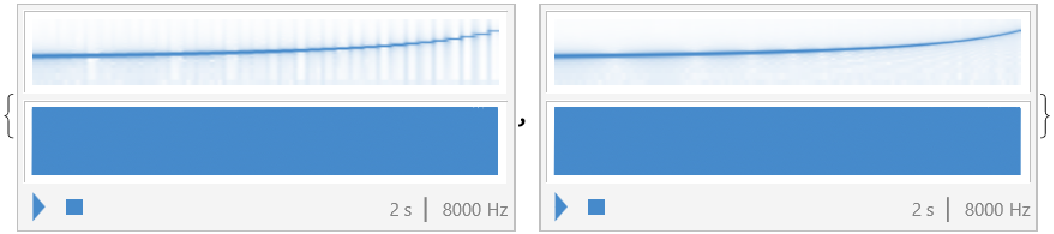### Properties and Relations (2)

Rescaling or adding a constant to the plotted function or the independent variable does not affect the output of FunctionAudiolize, as the latter automatically rescales the function to match the frequency range and audio duration criteria:

 In:=Out=FunctionAudiolize uses the built-in function Play to make sounds, so the output of FunctionAudiolize[1,{t,0,1},1] is exactly the same as that of Play[Sin[2π·440t],{t,0,1}]:

 In:=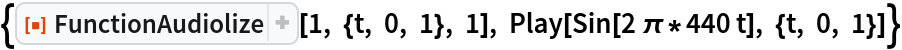Out=### Possible Issues (3)

The function does not accept complex numbers. Because of numerical errors, calculations that are supposed to yield real numbers sometimes end up returning something that has some imaginary part. The expression below is supposed to evaluate to 1+cos(x), but there also is a small imaginary error term:

 In:=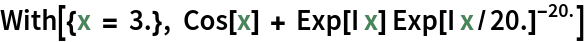Out=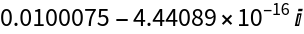Trying to audiolize this results in an error message:

 In:=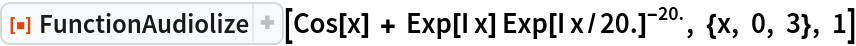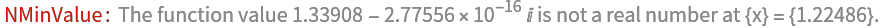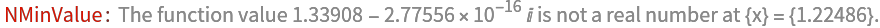Out=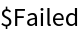If it is known for sure that the plotted function is real-valued, one can just plot the real part:

 In:=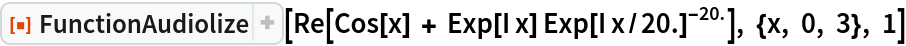Out=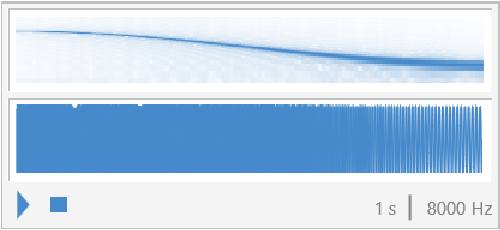### Neat Examples (5)

Audiolization of the Riemann-Siegel function:

 In:=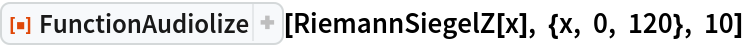Out=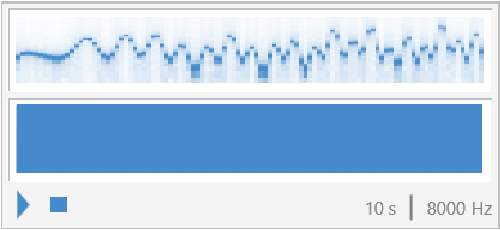The sound plot of the Fibonacci numbers mod 17, f(x)=FFloor[x]mod17, sounds like a pretty decent intro for piece of Prog Jazz:

 In:=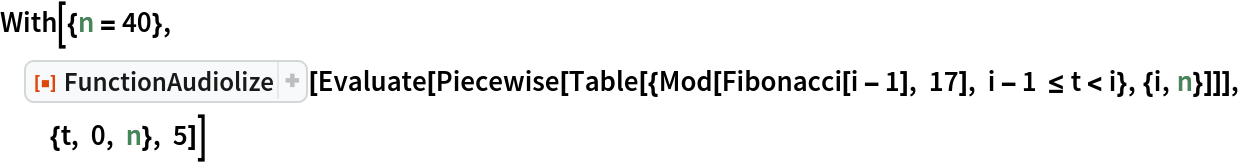Out=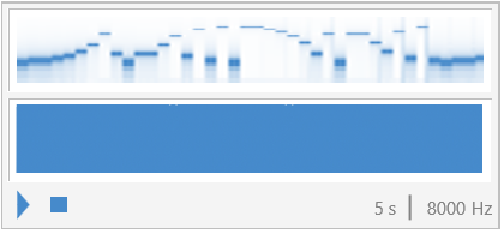Make a function whose audiolization is "The Last Airbender" theme:

 In:=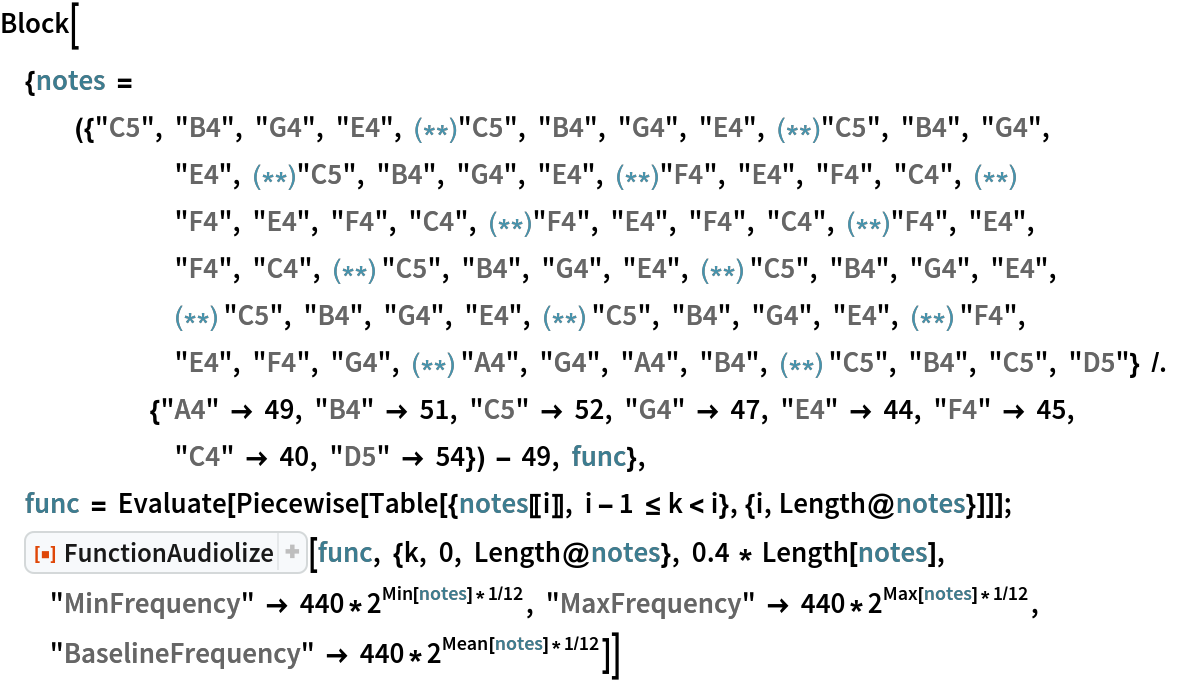Out=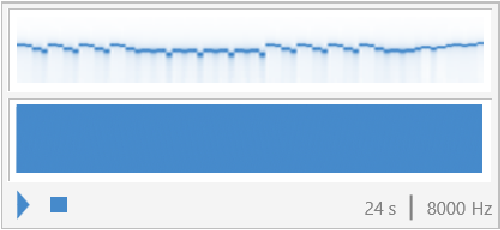The audiolization of log(log(x)) sounds like a death ray in the process of charging:

 In:=Out=Audiolization of an Airy function:

 In:=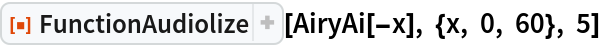Out=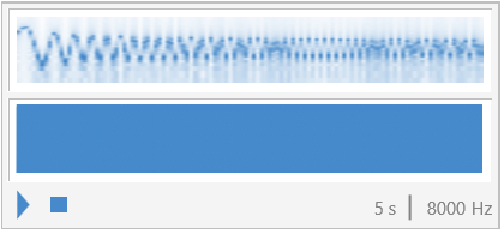## Publisher

Wolfram Summer Camp

## Version History

• 1.0.0 – 25 January 2022

## Author Notes

Despite all the potential applications, FunctionAudiolize is probably more of a just-for-fun curiosity than some sort of industrial pragmatic product. So have fun playing with it!